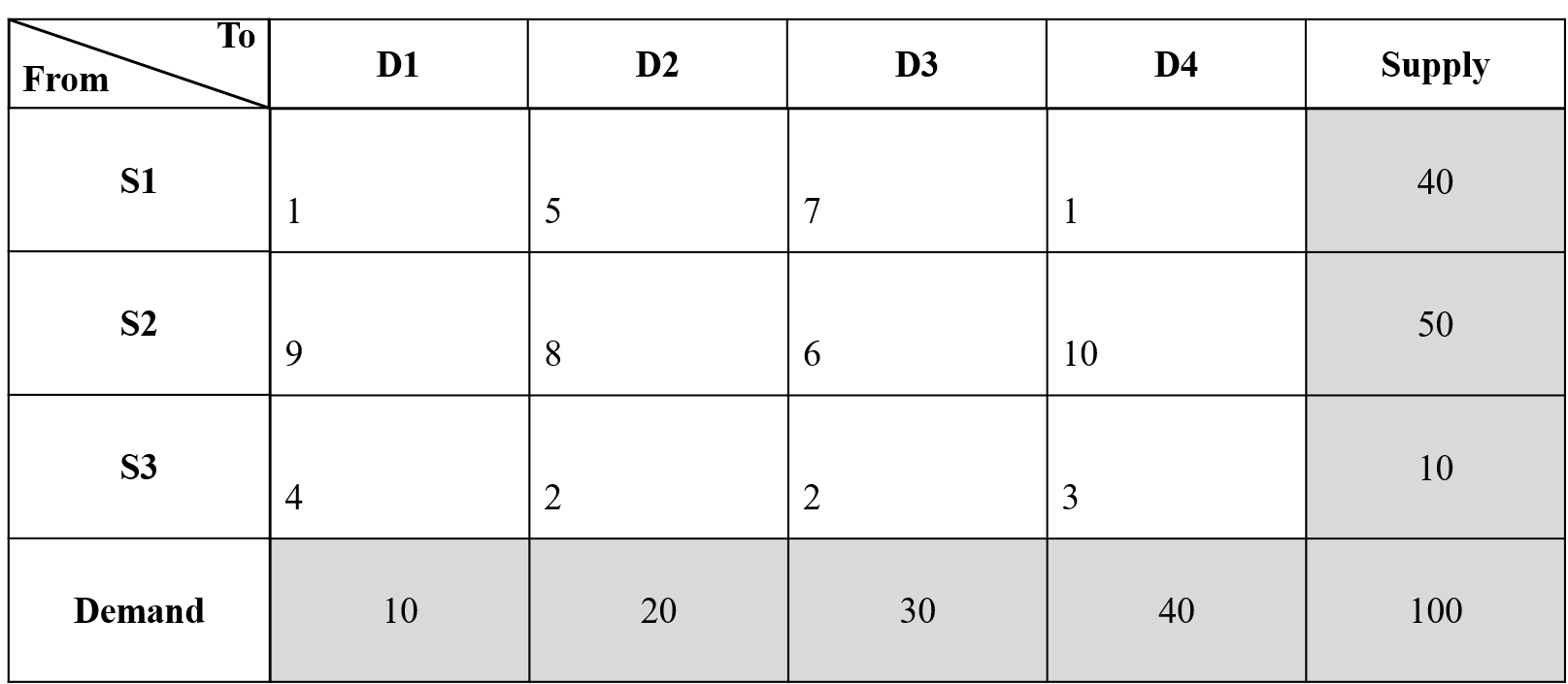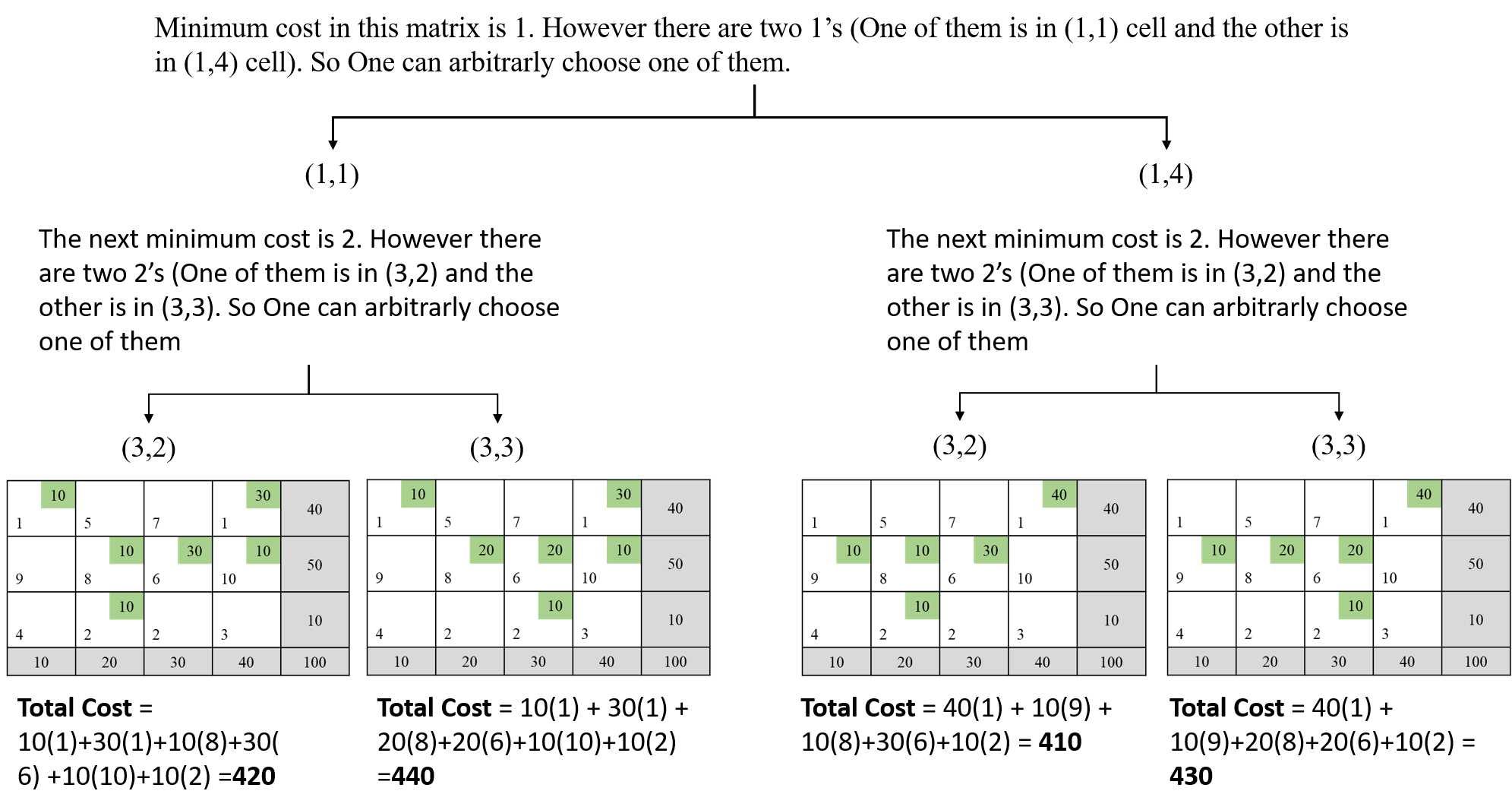# Problem 47623. Create initial basic feasible solution for transportation problems -Minimum Cost Method

Previous Problem : Problem 47618
Consider following transportation problem:You should return the assignment matrix where the total cost is minimum. In this case assignment matrix should be:
assignmentMatrix = [
0 0 0 40
10 10 30 0
0 10 0 0];
And total cost should be 410.

### Solution Stats

30.56% Correct | 69.44% Incorrect
Last Solution submitted on Sep 21, 2023

### Community Treasure Hunt

Find the treasures in MATLAB Central and discover how the community can help you!

Start Hunting!